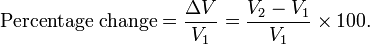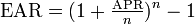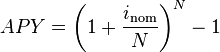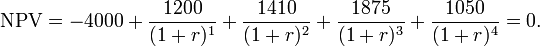## Calculating the Yield of a Single-Period Investment

The yield of a single period investment is simply $\frac { \left( \text{FV}\quad -\quad \text{PV} \right) }{ \text{PV} } \ast 100%.$.

### Learning Objectives

Differentiate between the different methods of calculating yield of a single period investment

### Key Takeaways

#### Key Points

• There are a number of ways to calculate yield, but the most common ones are to calculate the percent change from the initial investment, APR, and APY (or EAR).
• APR (annual percentage rate) is a commonly used calculation that figures out the nominal amount of interest accrued per year. It does not account for compounding interest.
• APY (annual percentage yield) is a way of using the nominal interest rate to calculate the effective interest rate per year. It accounts for compounding interest.
• EAR (effective annual rate) is a special type of APY that uses APR as the nominal interest rate.

#### Key Terms

• Effective Interest: The amount of interest accrued per year after accounting for compounding.
• Nominal Interest: The amount of interest accrued per year without accounting for compounding.
• effective-interest method: amortizing a debt according to the effective interest rate paid

### Determining Yield

The yield on an investment is the amount of money that is returned to the owner at the end of the term. In short, it’s how much you get back on your investment.

Naturally, this is a number that people care a lot about. The whole point of making an investment is to get a yield. There are a number of different ways to calculate an investment’s yield, though. You may get slightly different numbers using different methods, so it’s important to make sure that you use the same method when you are comparing yields. This section will address the yield calculation methods you are most likely to encounter, though there are many more.

### Change-In-Value

The most basic type of yield calculation is the change-in-value calculation. This is simply the change in value (FV minus PV) divided by the PV times 100%. This calculation measures how different the FV is from the PV as a percentage of PV.Percent Change: The percent change in value is the change in value from PV to FV (V2 to V1) divided by PV (V1) times 100%.

### Annual Percentage Rate

Another common way of calculating yield is to determine the Annual Percentage Rate, or APR. You may have heard of APR from ads for car loans or credit cards. These generally have monthly loans or fees, but if you want to get an idea of how much you will accrue in interest per year, you need to calculate an APR. Nominal APR is simply the interest rate multiplied by the number of payment periods per year. However, since interest compounds, nominal APR is not a very accurate measure of the amount of interest you actually accrue.

### Effective Annual Rate

To find the effective APR, the actual amount of interest you would accrue per year, we use the Effective Annual Rate, or EAR.EAR: The Effective Annual Rate is the amount of interest actually accrued per year based on the APR. n is the number of compounding periods of APR per year.

For example, you may see an ad that says you can get a car loan at an APR of 10% compounded monthly. That means that APR=.10 and n=12 (the APR compounds 12 times per year). That means the EAR is 10.47%.

The EAR is a form of the Annual Percentage Yield (APY). APY may also be calculated using interest rates other than APR, so a more general formula is in. The logic behind calculating APY is the same as that used when calculating EAR: we want to know how much you actually accrue in interest per year. Interest usually compounds, so there is a difference between the nominal interest rate (e.g. monthly interest times 12) and the effective interest rate.Annual Percentage Yield: The Annual Percentage Yield is a way or normalizing the nominal interest rate. Basically, it is a way to account for the time factor in order to get a more accurate number for the actual interest rate.inom is the nominal interest rate.N is the number of compounding periods per year.

## Calculating the Yield of an Annuity

The yield of an annuity is commonly found using either the percent change in the value from PV to FV, or the internal rate of return.

### Learning Objectives

Calculate the yield of an annuity using the internal rate of return method

### Key Takeaways

#### Key Points

• The yield of an annuity may be found by discounting to find the PV, and then finding the percentage change from the PV to the FV.
• The Internal Rate of Return (IRR) is the discount rate at which the NPV of an investment equals 0.
• The IRR calculates an annualized yield of an annuity.

#### Key Terms

• yield: In finance, the term yield describes the amount in cash that returns to the owners of a security. Normally it does not include the price variations, at the difference of the total return. Yield applies to various stated rates of return on stocks (common and preferred, and convertible), fixed income instruments (bonds, notes, bills, strips, zero coupon), and some other investment type insurance products
• Internal Rate of Return (IRR): The discount rate that will cause the NPV of an investment to equal 0.
• Net Present Value (NPV): The present value of a project or an investment decision determined by summing the discounted incoming and outgoing future cash flows resulting from the decision.

The yield of annuity can be calculated in similar ways to the yield for a single payment, but two methods are most common.

The first is the standard percentage-change method. Just as for a single payment, this method calculated the percentage difference between the FV and the PV. Since annuities include multiple payments over the lifetime of the investment, the PV (or V1 in is the present value of the entire investment, not just the first payment.

The second popular method is called the internal rate of return (IRR). The IRR is the interest rate (or discount rate) that causes the Net Present Value (NPV) of the annuity to equal 0. That means that the PV of the cash outflows equals the PV of the cash inflows. The higher the IRR, the more desirable is the investment. In theory, you should make investment with an IRR greater than the cost of capital.

Let’s take an example investment: It is not technically an annuity because the payments vary, but still is a good example for how to find IRR:

Suppose you have a potential investment that would require you to make a $4,000 investment today, but would return cash flows of$1,200, $1,410,$1,875, and \$1,050 in the four successive years. This investment has an implicit rate of return, but you don’t know what it is. You plug the numbers into the NPV formula and set NPV equal to 0. You then solve for r, which is your IRR (it’s not easy to solve this problem by hand. You will likely need to use a business calculator or Excel). When r = 14.3%, NPV = 0, so therefore the IRR of the investment is 14.3%.IRR Example: The setup to find the IRR of the investment with cash flows of -4000, 1200, 1410, 1875, and 1050. By setting NPV = 0 and solving for r, you can find the IRR of this investment.